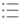### 获取当前时间戳

 Swift ``NSDate().timeIntervalSince1970`` Go ``````import ( "time" ) int32(time.Now().Unix())`````` Java ``````// pure java (int) (System.currentTimeMillis() / 1000)`````` ``````// joda (int) (DateTime.now().getMillis() / 1000)`````` JavaScript ``Math.round(new Date() / 1000)`` Objective-C ``[[NSDate date] timeIntervalSince1970]`` MySQL ``SELECT unix_timestamp(now())`` SQLite ``SELECT strftime('%s', 'now')`` Erlang ``calendar:datetime_to_gregorian_seconds(calendar:universal_time())-719528*24*3600.`` PHP ``````// pure php time()`````` ``````// Carbon\Carbon Carbon::now()->timestamp`````` Python ``````import time time.time()`````` Ruby ``Time.now.to_i`` Shell ``date +%s`` Groovy ``(new Date().time / 1000).intValue()`` Lua ``os.time()`` .NET/C# ``(DateTime.Now.ToUniversalTime().Ticks - 621355968000000000) / 10000000``